## 3.7.1 Simple Model

Example 12: Design of a Response Distfict

Suppose that we have once more the situation described in Exercise 3.1, where requests for assistance are medical emergencies and the urban response unit is an ambulette. Under the assumptions that (1) locations of a medical emergency (X1, Y1) and of the ambulette (X2, Y2) are independent and uniformly distributed over the response district, and (2) travel is parallel to the sides of the rectangular response area, the travel distance [from (3.11)] is given by

D = |X1 - X2| + |Y1 - Y2|

From Exercise 3.1, we then have that

E[D) = 1/3[Xo + YJ             (3.12a)

where X0 and Y0 are the sides of the rectangle (see Figure 3.3). In this example we wish to formulate and solve the problem of optimal district design and to investigate the sensitivity of our results to suboptimal designs.

Solution

To find the district dimensions which lead to the minimum expected travel distance, we must keep the area of the response district
A0 = X0 Y0 constant and minimize (3.12a) subject to the condition YO = AO/XO. Without this constant, a zero area (point) district would be optimal, an obviously infeasible result considering that the collection of districts in a city must usually cover the entire city (which has fixed positive area). Not surprisingly, (3.12a) is minimized when the rectangle becomes a square,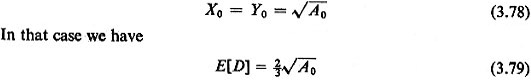More generally, if the effective travel speeds in the x-direction and the y-direction, vx and vy, are independent of travel distance, the expected travel time,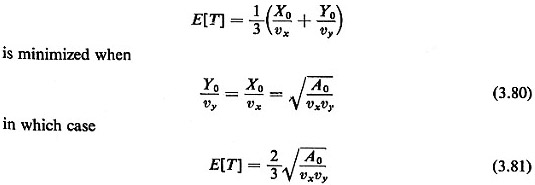Intuitively speaking, the optimal shape of the district, as given by (3.80), is the one for which it takes as much time to traverse the district from "east to west" as from "north to south."
The expressions for E[D] and E[T] turn out to be "robust" (i.e., rather insensitive to the exact values of X0 and Y0). To see this, let us examine the case where

X0 =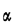Y0                          (3.82)

whereis a positive constant. Without loss of generality we assume> 1 and, as before, we set A0 = X0 Y0. Then (3.12) can be written as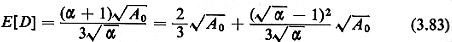The second term in (3.83) is the amount by which E[D] deviates from its minimum value in (3.79). For= 1.5 that term becomes equal to 0.014,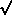A0 (i.e., E[D] is only about 2 percent greater than its minimum value). Even for= 4, E[D] is only 25 percent more than its minimum value. An entirely similar analysis can demonstrate the robustness of (3.80).

Results such as those of (3.79) and (3.80) can be derived for various district shapes. The first three columns of Table 3-1 summarize the equivalents of (3.79) for a square district, a square district rotated by 45' with respect to the right-angle directions of travel, and a circular district. The following four cases are included:8

1. Euclidean (straight-line) travel when the response unit is randomly and uniformly positioned in the district.
2. Case I with right-angle travel.
3. Euclidean travel with the response unit located at the center of the district.
4. Case 3 with right-angle travel.

In all cases it is assumed that the locations of requests for service are uniformly distributed in the district and independent of the location of the service unit. When the constants in Table 3-1 are multiplied by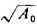, the square root of the area of the district in question, E[D] is obtained. In some instances (e.g., a square district with a randomly positioned response unit and Euclidean travel) the constant of interest is not known exactly and the best known approximation, to two-decimal-place accuracy, is shown. Some of these constants have already been derived in this chapter or will be derived in the Problems.The three district geometries included in Table 3-1 are "special cases" of rectangular, diamond-shaped, and elliptic districts. If one varies the district dimensions of each type while constraining district area to equal a constant A0, E[D] is minimized by the symmetric geometries represented in Table 3-1.
It can be seen from Table 3-1 that, for any given district area A, E[D] is very insensitive to the exact geometry of the district. This can be confirmed by deriving E[D] for other possible district geometries, such as equilateral triangles or piece-of-pie-like sectors of circles. Moreover, for any given district geometry, the value of E[D] is insensitive to changes of the dimensions of the district that might make it appear to deviate appreciably from its optimum shape. This, too, can be confirmed by performing a sensitivity analysis similar to the one for the rectangular district in Example 12.
From these observations it can be concluded that we can use the first three columns of Table 3-1 to infer similar approximate expressions for E[D] that apply to districts of any shape as long as (1) one of the dimensions (e.g., "length") is not much greater than the other dimension (e.g., width), and (2) major barriers or boundary indentations do not exist in the district. Districts that satisfy both of the conditions above will be called here, informally, "fairly compact and fairly convex districts." We can now state the following:

For fairly compact and fairly convex districts and for independently and spatially uniformly distributed requests for service,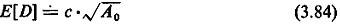where A0,, is the area of the district and c is a constant that depends only on the metric in use and on the assumption regarding the location of the response unit in the district.

The last column of Table 3-1 lists values that can be used for c in (3.84) for the four combinations of response unit locations and metrics that we have examined here. In all cases, we have selected the largest value of c listed in each row of the three leftmost columns of Table 3-1.
When the effective travel speed is independent of the distance covered, one can use the constants in the fourth column of Table 3-1 to approximate the expected travel time, E[T), as well. In that case we have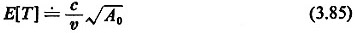in the case of Euclidean travel (assuming that the effective travel speed v is independent of the direction of travel) and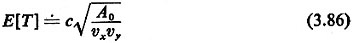for right-angle travel. In this latter case, the district "compactness" statement requires that

E[Teast-west]E[Tnorth-south]

That is, it takes on the average about as much time to traverse the district from east to west as from north to south.
Another observation that can be made on the basis of the foregoing discussion is that both E[D] and E[T] are proportional to the square root of the district area, A0,, irrespective of the specific distance metric in use. This is hardly surprising since this relationship is basically a dimensional one: distance is the square root of area. More formally, if the coordinates of each point (x, y) in the district of interest are multiplied bym (m > 1) [i.e., point (x, y) now becomes point (m x,m y)], then the area of the district increases m-fold but the length, L, of any given route between the pair of points (x1, y1,) and (x2,, y2,) in the original district-becomes equal tomL in the expanded district.
Equivalently, we can state that E[D] and E[Tj must be proportional to the inverse of the square root of the density of response units in a district, for districts with more than one response unit. That is, if a district of area A is divided into n approximately equal fairly convex and fairly compact subdistricts of responsibility (whose shapes may vary), then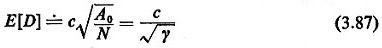where y denotes the spatial density of service units. We shall derive the same functional type of relationship in a somewhat different context later in this chapter [cf. (3.101a) and (3.104a)].

8 few results for metrics other than Euclidean or right-angle are derived in the Problems.